##### 一维数组

``````type_t arr_name [const_n]
//type_t:数组元素类型。arr_name数组名。const_n常量表达式，指定数组大小。
int arr ;``````

``int arr = {1，2，3}  //其余元素默认初始化为0``

::: warning

``````int arr1 = {'a','b'};  //数组中只有a、b两个元素，其余默认初始化为0
int arr2 = "ab";  //数组中有a、b、\0、三个元素，其余默认初始化为0``````

``char arr  = {a,98};  ``

``char arr[] = "abcdefg";``

###### sizeof和strlen
``````#include <stdio.h>
#include <string.h>

int main()
{
char arr[] = "abcdefg";
printf("sizeof(arr)=%d\n", sizeof(arr));
printf("strlen(arr)=%d\n", strlen(arr));
return 0;
}``````
``````sizeof(arr)=8
strlen(arr)=7``````

::: warning sizeof是运算符，是计算数组所占空间的大小（包括数组内元素和标识结尾的‘\0’），即n*1+1。 strlen是库函数，测量的是字符串的实际长度，以'\0'结束（不包括'\0'），即n。

strlen：只能针对字符串求长度 sizeof：计算变量、数组、类型的大小，单位是字节。 :::

``````#include <stdio.h>
#include <string.h>

int main()
{
//char arr[] = "abcdefg";
//printf("%d\n", sizeof(arr));
//printf("%d\n", strlen(arr));
char arr1[] = "abcde";
char arr2[] = { 'a','b','c','d','e' };
printf("sizeof(arr1)=%d\n", sizeof(arr1));
printf("sizeof(arr2)=%d\n", sizeof(arr2));
printf("strlen(arr1)=%d\n", strlen(arr1));
printf("strlen(arr2)=%d\n", strlen(arr2));
return 0;
}``````
``````sizeof(arr1)=6
sizeof(arr2)=5
strlen(arr1)=5
strlen(arr2)=34``````

``````#include <stdio.h>

int main()
{
char arr1[] = "abcde";
char arr2[] = { 'a','b','c','d','e' };
char arr3 = { 'a','b','c','d','e' };
return 0;``````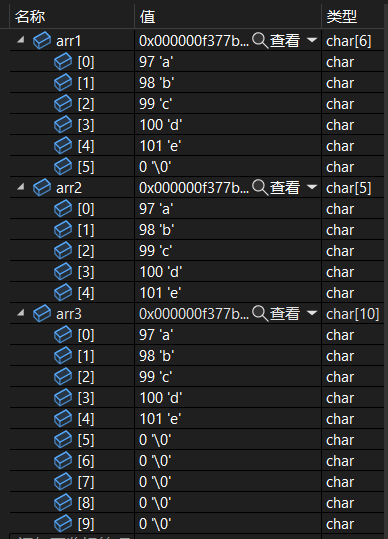###### 数组的访问

``````#include <stdio.h>

int main()
{
char arr[] = { 'a','b','c','d','e','f'};
int i = 0;
for (i; i < 3; i++)
{
printf("%c", arr[i]);
}
return 0;
}``````
``abc``

``````#include <stdio.h>
#include <string.h>

int main()
{
char arr1[] = "abcdefg";
int len = strlen(arr1);
printf("%d\n", len);
int n = 0;
for (n; n < len; n++)
{
printf("%c", arr1[n]);
}
return 0;
}``````
``````7
abcdefg``````

``````#include <stdio.h>

int main()
{
int arr2[] = { 1,2,3,4,5,6,7,8,9 };
int sz = sizeof(arr2) / sizeof(arr2);
printf("%d\n", sz);
int n = 0;
for (n; n < sz; n++)
{
printf("%d", arr2[n]);
}
return 0;
}``````
``````9
123456789``````

::: danger 数组元素写成""形式时，数组中包含“\0”。 数组元素写成{}形式时，数组中不包含“\0”。 使用strlen计算数组长度时，从首元素到“\0”为止，不包含“\0”。 使用sizeof计算数组长度时，包括数组内元素和标识结尾的‘\0’。 :::

###### 一维数组的内存存储

``````#include <stdio.h>

int main()
{
int arr[] = { 0,1,2,3,4,5,6,7,8,9 };
int sz = sizeof(arr) / sizeof(arr);
int i = 0;
for (i; i < sz; i++)
{
printf("元素%d的地址为%p\n", i, &arr[i]);
}
return 0;
}``````
``````元素0的地址为0000000ACA2FF648

##### 二维数组

``````int arr[x][y];
char arr[x][y];
double arr[x][y];``````
###### 二维数组的初始化
``  int arr = { 1,2,3,4,5,6,7,8,9 };``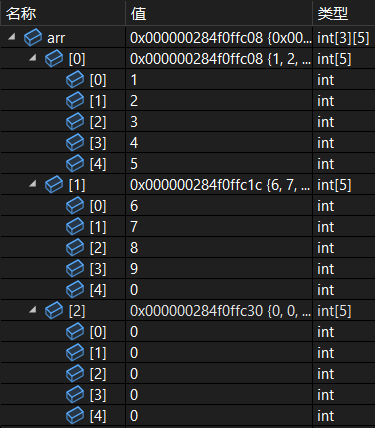由上可得二维数组的元素填充是先行后列，其余未赋值元素默认初始化为0。 二维数组可以指定初始化元素的位置：

``int arr = { {1,2,3,4},{5,6},7,8,9 };``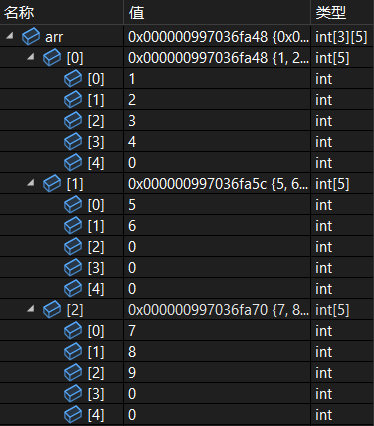``int arr[] = { {1,2,3,4},{5,6} };``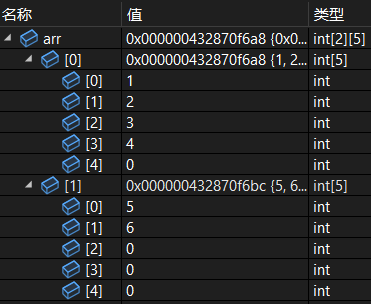::: warning 二维数组的行和列不能同时省略。 二维数组行可以省略，列不能省略。 :::

###### 二维数组的使用

``````#include <stdio.h>

int main()
{
int arr = { {1,2,3,4},{5,6} };
int m = 0;
for (m; m < 2; m++)
{
int n = 0;
for (n; n < 5; n++)
{
printf("%d",arr[m][n]);
}
printf("\n");
}
return 0;
}``````
``````12340
56000``````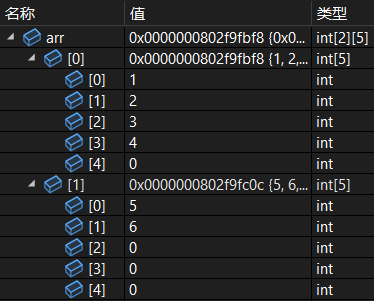::: tip 二维数组和一维数组一样通过下标访问。 :::

###### 二维数组的内存存储
``````#include <stdio.h>

int main()
{
int arr = { {1,2,3,4},{5,6} };
int m = 0;
for (m; m < 2; m++)
{
int n = 0;
for (n; n < 5; n++)
{
printf("&arr[%d][%d]=%p\n",m,n,&arr[m][n]);
}
}
return 0;
}``````
``````&arr=0000004F8DCFF868
&arr=0000004F8DCFF86C
&arr=0000004F8DCFF870
&arr=0000004F8DCFF874
&arr=0000004F8DCFF878
&arr=0000004F8DCFF87C
&arr=0000004F8DCFF880
&arr=0000004F8DCFF884
&arr=0000004F8DCFF888
&arr=0000004F8DCFF88C``````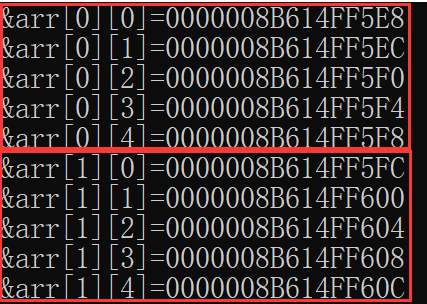由上图可知，二维数组中每一行的元素地址相差4个字节，第一行和第二行元素也相差4个字节。即二维数组在内存中也是连续存放的。二维数组本质是由一维数组组成的。Suzhou
Lv1

17

11

0